# NCERT Solutions for Class 8 Maths Chapter 9 Algebraic Expressions and Identities Ex 9.5

NCERT Solutions for Class 8 Maths Chapter 9 Algebraic Expressions and Identities Exercise 9.5

Ex 9.5 Class 8 Maths Question 1.
Use a suitable identity to get each of the following products:
(i) (x + 3) (x + 3)
(ii) (2y + 5) (2y + 5)
(iii) (2a – 7) (2a – 7)
(iv) (3a – $$\frac { 1 }{ 2 }$$) (3a – $$\frac { 1 }{ 2 }$$)
(v) (1.1m – 0.4) (1.1m + 0.4)
(vi) (a2 + b2) (-a2 + b2)
(vii) (6x – 7) (6x + 7)
(viii) (-a + c) (-a + c)
(ix) ($$\frac { x }{ 2 }$$ + $$\frac { 3y }{ 4 }$$) ($$\frac { x }{ 2 }$$ + $$\frac { 3y }{ 4 }$$)
(x) (7a – 9b) (7a – 9b)
Solution: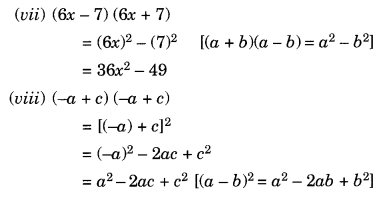Ex 9.5 Class 8 Maths Question 2.
Use the identity (x + a)(x + b) = x2 + (a + b)x + ab to find the following products.
(i) (x + 3) (x + 7)
(ii) (4x + 5)(4x + 1)
(iii) (4x – 5) (4x – 1)
(iv) (4x + 5) (4x – 1)
(v) (2x + 5y) (2x + 3y)
(vi) (2a2 + 9) (2a2 + 5)
(vii) (xyz – 4) (xyz – 2)
Solution:Ex 9.5 Class 8 Maths Question 3.
Find the following squares by using the identities.
(i) (b – 7)2
(ii) (xy + 3z)2
(iii) (6x2 – 5y)2
(iv) ($$\frac { 2 }{ 3 }$$ m + $$\frac { 3 }{ 2 }$$ n)2
(v) (0.4p – 0.5q)2
(vi) (2xy + 5y)2
Solution: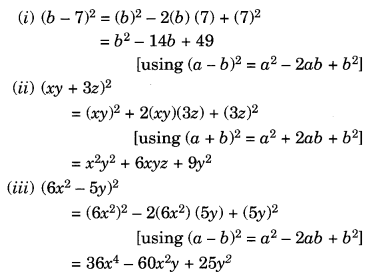Ex 9.5 Class 8 Maths Question 4.
Simplify:
(i) (a2 – b2)2
(ii) (2x + 5)2 – (2x – 5)2
(iii) (7m – 8n)2 + (7m + 8n)2
(iv) (4m + 5n)2 + (5m + 4n)2
(v) (2.5p – 1.5q)2 – (1.5p – 2.5q)2
(vi) (ab + bc)2 – 2ab2c
(vii) (m2 – n2m)2 + 2m3n2
Solution: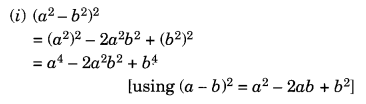Ex 9.5 Class 8 Maths Question 5.
Show that:
(i) (3x + 7)2 – 84x = (3x – 7)2
(ii) (9p – 5q)2 + 180pq = (9p + 5q)2
(iii) ($$\frac { 4 }{ 3 }$$ m – $$\frac { 3 }{ 4 }$$ n)2 + 2mn = $$\frac { 16 }{ 9 }$$ m2 + $$\frac { 9 }{ 16 }$$ n2
(iv) (4pq + 3q)2 – (4pq – 3q)2 = 48pq2
(v) (a – b)(a + b) + (b – c) (b + c) + (c – a) (c + a) = 0
Solution: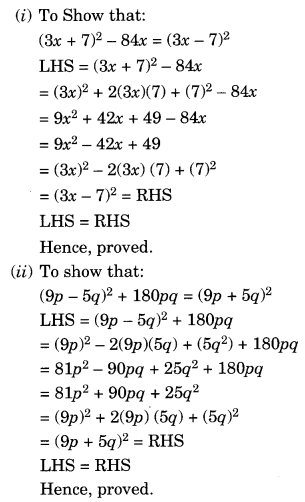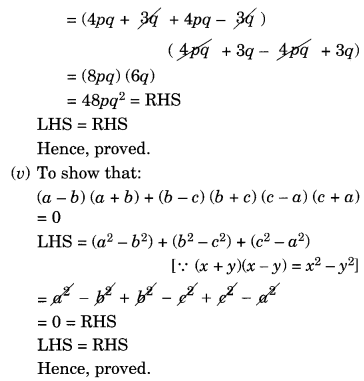Ex 9.5 Class 8 Maths Question 6.
Using identities, evaluate:
(i) 712
(ii) 992
(iii) 1022
(iv) 9982
(v) 5.22
(vi) 297 × 303
(vii) 78 × 82
(viii) 8.92
(ix) 1.05 × 9.5
Solution:Ex 9.5 Class 8 Maths Question 7.
Using a2 – b2 = (a + b) (a – b), find
(i) 512 – 492
(ii) (1.02)2 – (0.98)2
(iii) 1532 – 1472
(iv) 12.12 – 7.92
Solution:
(i) 512 – 492 = (51 + 49) (51 – 49) = 100 × 2 = 200
(ii) (1.02)2 – (0.98)2 = (1.02 + 0.98) (1.02 – 0.98) = 2.00 × 0.04 = 0.08
(iii) 1532 – 1472 = (153 + 147) (153 – 147) = 300 × 6 = 1800
(iv) 12.12 – 7.92 = (12.1 + 7.9) (12.1 – 7.9) = 20.0 × 4.2 = 84

Ex 9.5 Class 8 Maths Question 8.
Using (x + a) (x + b) = x2 + (a + b)x + ab, find
(i) 103 × 104
(ii) 5.1 × 5.2
(iii) 103 × 98
(iv) 9.7 × 9.8
Solution:
(i) 103 × 104 = (100 + 3)(100 + 4) = (100)2 + (3 + 4) (100) + 3 × 4 = 10000 + 700 + 12 = 10712
(ii) 5.1 × 5.2 = (5 + 0.1) (5 + 0.2) = (5)2 + (0.1 + 0.2) (5) + 0.1 × 0.2 = 25 + 1.5 + 0.02 = 26.5 + 0.02 = 26.52
(iii) 103 × 98 = (100 + 3) (100 – 2) = (100)2 + (3 – 2) (100) + 3 × (-2) = 10000 + 100 – 6 = 10100 – 6 = 10094
(iv) 9.7 × 9.8 = (10 – 0.3) (10 – 0.2) = (10)2 – (0.3 + 0.2) (10) + (-0.3) (-0.2) = 100 – 5 + 0.06 = 95 + 0.06 = 95.06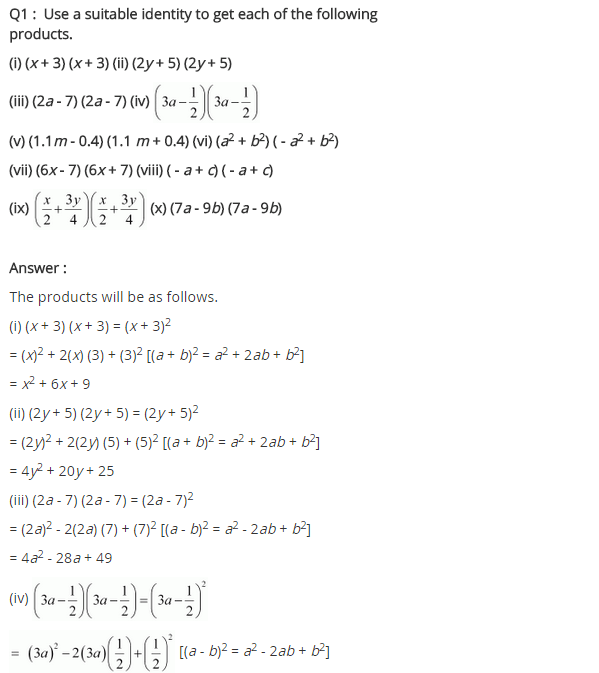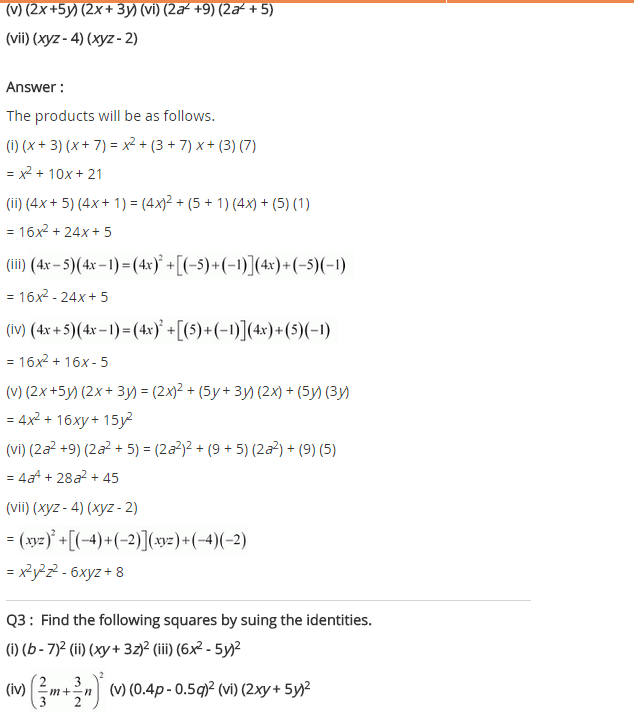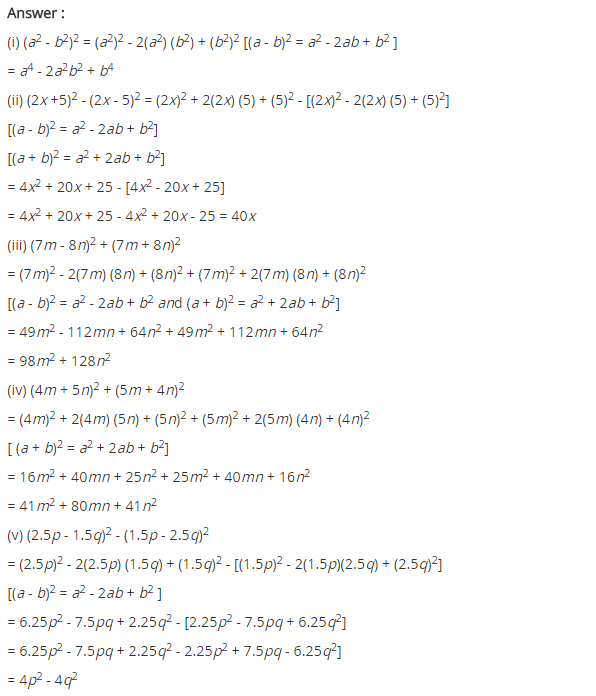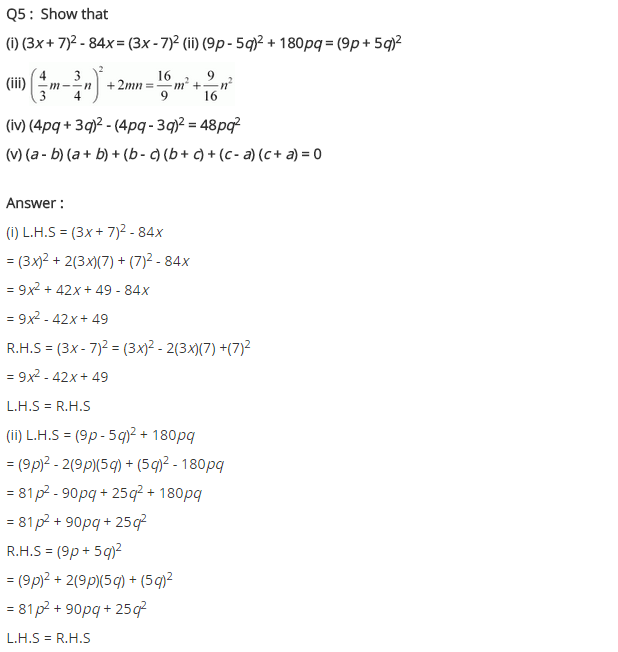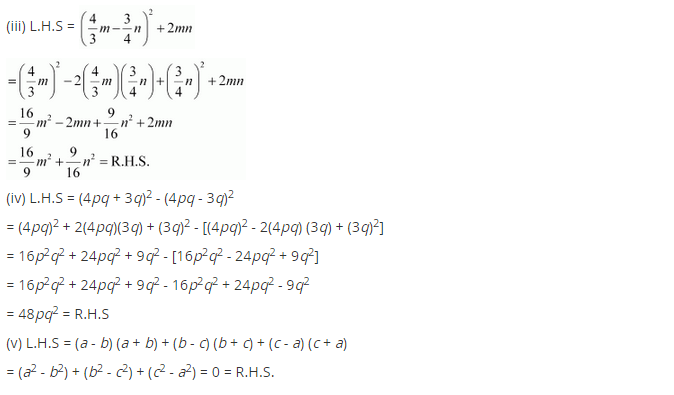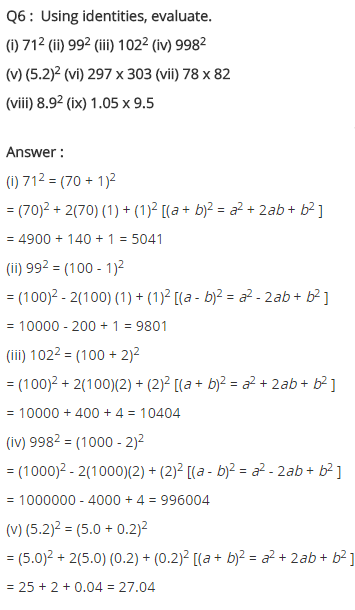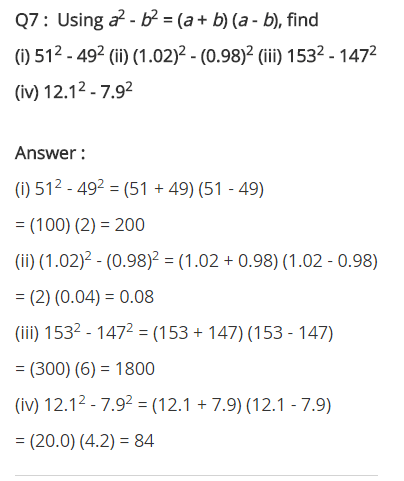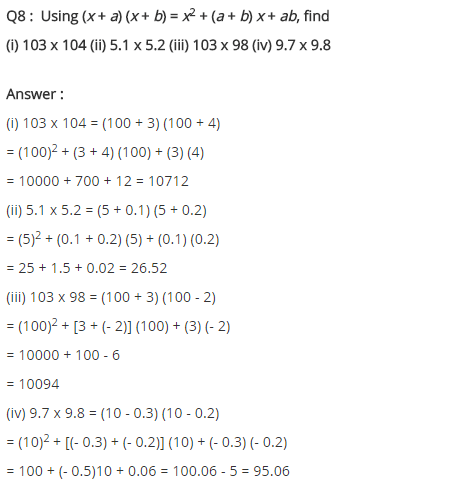+